# Applying Automatic Drift Correction to Mass Spectrometry

The ability to measure mass to charge ratio of the ions in mass spectrum is the most important feature of the high-resolution mass spectrometers. An analyte's identification can be progressed further as sufficient mass accuracy allows the unique assignment of elemental formula.

An example is given in Figure 1 to demonstrate how the number of possibly assigned elemental compositions is dependent on the available measured mass accuracy for that particular instrument and sample. It clearly shows that the number of possible hits is drastically reduced with lower (i.e. better) mass accuracy values and selection for the accurate analyte assignment is narrowed down.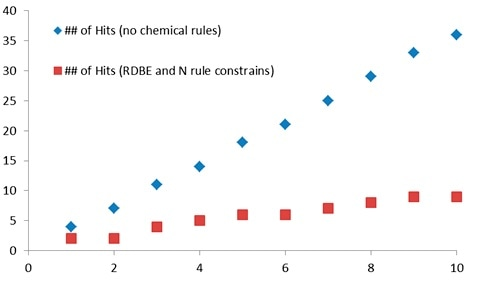Figure 1. Example of the number of possible elemental compositions of the compound with m/z=276.09327 vs mass error tolerance (Cc Hh Oo Nn Ss Pp c=1-30, h=1-30, o=0-8, n=0-5, s=0-4, p=0-2).

## Calculations of the Mass Accuracy

The mass accuracy is typically measured in millidaltons (mDa) or parts-per-million (ppm). Mass accuracy calculations can be carried out using the following formulae:

MA=(Mm-Mt)*1000, [mDa]           (1)
MA=((Mm-Mt)/ Mt)*106, [ppm],    (2)

where Mm is measured m/z of the ion of interest and Mt is theoretically calculated m/z based on the elemental composition of the ion of interest.

With the example in Figure 2, a measured value of m/z Mm =276.09327 is shown for the molecular ion in the mass spectrum of benzo(ghi)perylene. Using the ChromaTOF-HRT Mass Calculator tool, the theoretically calculated value for C22H12+ is Mt =276.09335. Using formulae (1) and (2), the resulted mass accuracy is calculated respectively as -0.08 mDa or -0.29 ppm.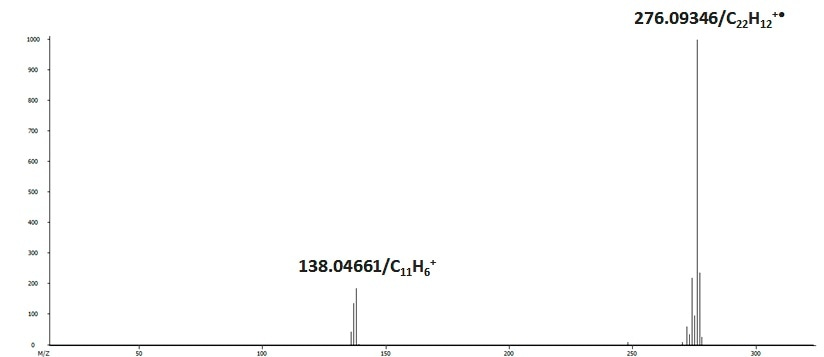Figure 2. The mass spectrum of benzo(ghi)perylene (C22 H11 ) acquired on GC-HRT.

## Sources of Mass Accuracy Errors

Mass measurement errors can occur through several sources. These include Mass Spectrometer resolving power; Mass Calibration errors; time-of-flight (TOF) drift of the ions during sample run and between runs; ion peaks TOF shifts due to signal saturation; and poor statistics, which therefore increases the uncertainty of the ion peaks TOF measurements from low ion signal.

This article addresses the ion TOF drift and applies methods of automatic drift correction to improve the mass accuracy of the data.

## Mass Calibration Procedures

The provision of adequate mass calibration is the very first step to achieving good mass accuracy. Using information about known ions in mass spectrum of the calibration compound, the mass calibration is carried out. As shown in Figure 3, in this case the compound is Perfluotributylamine (PFTBA, C12F27N). Second-order polynomial functions are fitted to the TOF – m/z pairs data from the known PFTBA ions in the acquired mass spectrum to define the mass calibration function coefficients C0, C1 and C2, as shown in Figure 4.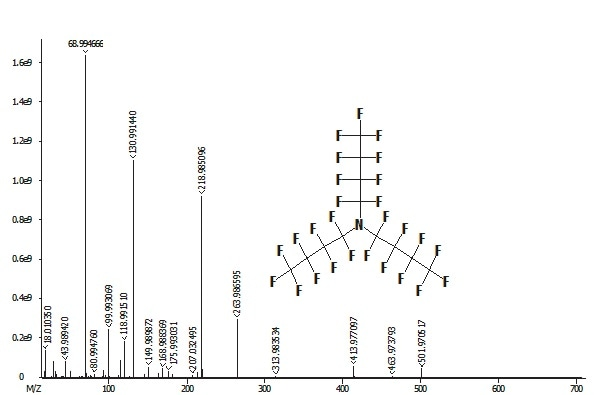Figure 3. PFTBA electron Ionization mass spectrum, obtained on GC-HRT.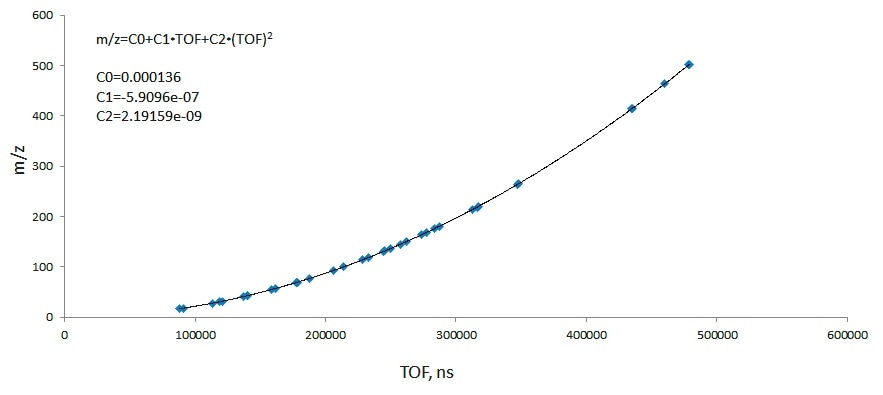Figure 4. Plot m/z vs TOF for the calibrant (PFTBA) ions and the second order polynomial curve fitting the data. The calibration function m/z=f(TOF) is defined from the second-order polynomial curve fit.

Before starting the analysis, the external mass calibration can be performed as the automated Mass Calibration routine. The mass calibration coefficients are stored with the respective MS method and applied automatically during data acquisition to convert ion TOFs to their respective m/zs.

Already collected data is used for the internal mass calibration, which is performed as a part of the Data Processing routine. Any known ion types can be included in the mass calibration matrix: PFTBA ions, persistent background ions (chemical background, column bleeds), and sample ions.

The Mass Calibration Table view in Figure 5 details the results of the standard (external or internal) calibration routines.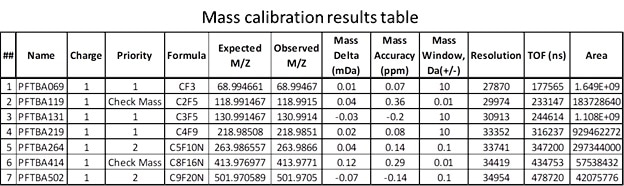Figure 5. Mass Calibration Table with all reported mass accuracy values for the calibrant and check masses well below 1 ppm.

Mass Calibration routine is performed on mass spectra. These are then summed over some time. The averaged TOF data is used to calculate the mass calibration coefficients under the assumption that TOF changes over that period are minimal. However, if a significant change is observed in the TOF of ions for some reason (for example, TOF drift several nanoseconds due to electrical or dimensional changes in the mass analyzer) then to achieve satisfactory mass accuracy values (<1 ppm) in the data standard mass calibration routine will not be enough.

The TOF Correction option is included with the Data Processing routine to address this problem. This can be performed on the collected data. The lock mass correction in this routine is performed on each spectrum and calibration correction coefficients are saved with the data.

## Automatic Drift Correction

Automatic TOF correction is provided in real-time throughout data acquisition with the ChromaTOF software – Automatic Drift Correction (ADC). The presence of persistent ion peaks in spectra are relied upon for this routine - background ions and/or ions from the PFTBA calibrant, which is typically co-introduced with the sample. Outstanding mass accuracy in the GC-HRT data is provided with the ADC, as shown in Figure 6. The following steps are performed by ChromaTOF software during the real-time ADC application:

• An on-the-fly list of the detected m/z is generated
• m/z which are considered unacceptable for use with ADC are removed from the list of ion peaks. For example, saturated, too low intensity, too low m/z, etc.
• The signal stability of the qualified ion peaks is evaluated over a certain period (for example, 15 spectra), where m/z area and peak width variations are required to be within an acceptable range
• An initial list of m/z is considered as a reference spectrum
• A new spectrum is considered after a set period and the software identifies any m/z's correction pairs. These are pairs of m/z's from the incoming spectrum and the reference spectrum.
• ADC picks the best pairs of ion peaks:
• Ion peaks with high intensity, most stable areas and stable widths are chosen preferentially
• A maximum of 30 ion peak pairs are used by ADC for calculations
• A drift correction slope is generated for each pair of ion peaks (reference and incoming) and the robust weighted (by area) average of these slopes is computed.
• The drift correction is the resulting average
• The corrected TOFs are calculated by multiplying this drift correction by the TOFs in the original (unfiltered) spectrum

The reference snapshot is corrected using the reference snapshot from the previous acquisition, which corrects drift between and within each acquisition.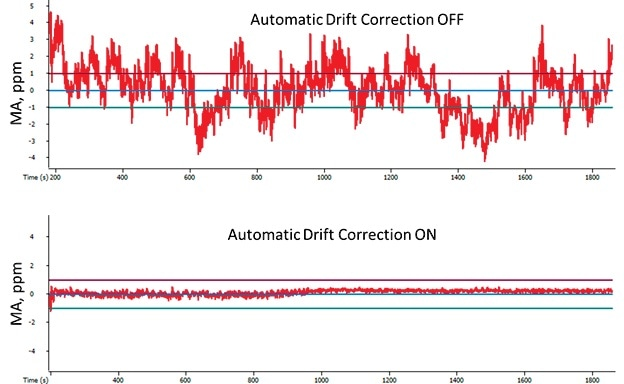Figure 6. Mass Accuracy for m/z 218.98501 (PFTBA fragment ion, C4F9+) measured during 30 min acquisition with ADC OFF (top) and ADC ON (bottom).

## Examples of the Mass Accuracy Improvement While Applied Automatic Drift Correction

The two following examples illustrate the importance of the ADC implementations.

Example 1. Figure 7 illustrates the TIC and m/z=276 chromatogram plots of the standard mixture data. The Benzo[ghi]perylene (C22H12, m/z=276.093352) is the part of this mixture. With the ADC ON and ADC OFF, the data was collected and the corresponding mass accuracy plots for the PFTBA fragment ion C4F9+ with m/z=218.985080, as shown in Figure 8. During the whole run, the PFTBA was co-introduced with the sample.

Correct analyte assignment has been made using the automatic peak finding procedure of the ChromaTOF software with mass accuracy error 0.04 ppm and data collected with ADC ON. The data processing of the sample, re-acquired with ADC OFF, resulted in the wrong analyte assignment: the proposed analyte with the minimal mass error of 0.88 ppm was N4,N4-dimethyl-2-(phenylsulfonyl)-1,4-benzenediamine (C14H16N2O2S, m/z=276.092700), and the m/z of the correct analyte (Benzo[ghi]perylene) was off by -3.24 ppm (Figure 9). Therefore, ADC implementation supported the reduction of the mass accuracy error and allowed for the correct analyte assignment.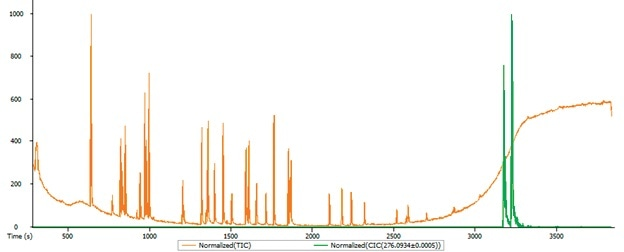Figure 7. The test mixture chromatograms: TIC – red, m/z=276.0934 – green.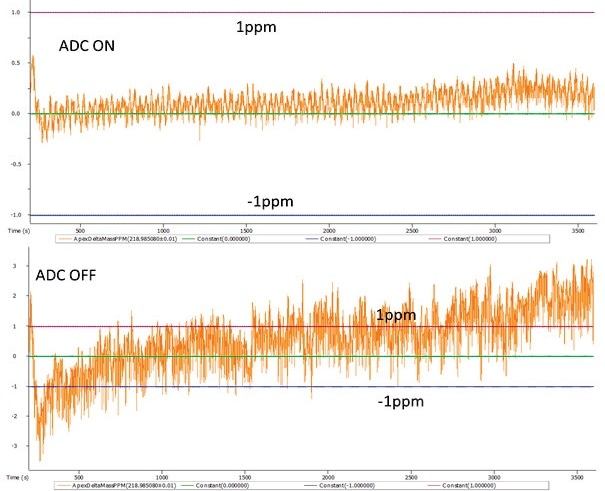Figure 8. Mass accuracy plots for the PFTBA fragment ion C4F9+ while analyzing test mixture with ADC ON (top) and ADC OFF (bottom).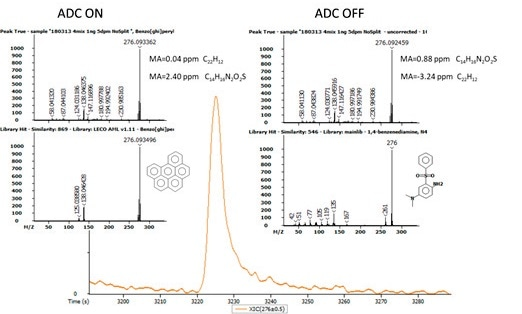Figure 9. The results of the automatic analyte assignment based on accurate mass formula calculation: correct with ADC ON (left) and wrong with ADC OFF (right).

Example 2. Mass accuracy can still be provided by the GC-HRT hardware in many cases even without ADC implementation. This is often considered good enough for other vendors' high-resolution TOFMS. However, user confidence in the automatically reported results will be reduced with higher mass error.

The test mixture chromatogram is shown in Figure 10 with overlaid m/z=152.0620 ion chromatogram, corresponding to the molecular ion of acenaphtylene (C12H8), which is a part of the mixture. The automatic analytes assigning results (Figure 11) are basically the same with ADC ON or OFF – if the accepted mass error window is set to 5 ppm then the acenaphtylene is correctly assigned in the both sets of data.

However, if a narrower mass error window is set, for example 2 ppm as recommended for analysis of complex mixtures, then the automatic peak assignment routine will not even include the correct analytes in the hit list while using accurate mass criteria. What’s more, the major advantage factor for analysis using high-resolution mass spectrometry is the option to apply high-mass accuracy criteria for analyte assignment.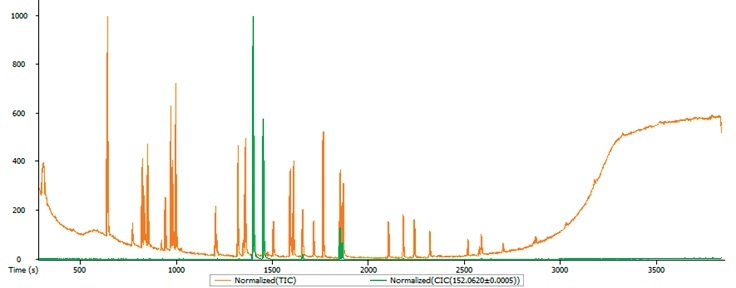Figure 10. The test mixture chromatograms: TIC – red, m/z=152.0620 – green.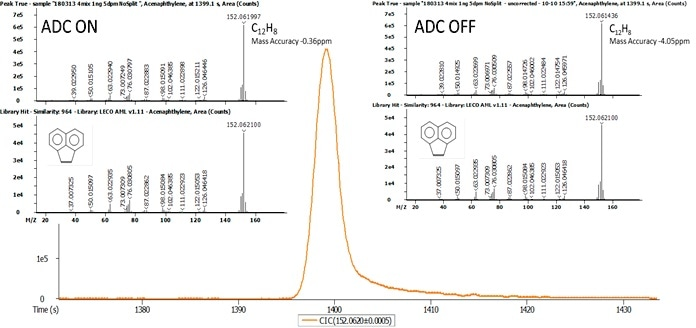Figure 11. The results of the automatic analyte assignment based on accurate mass formula calculation: mass error -0.36 ppm with ADC ON (left) and mass error -4.05 ppm with ADC OFF (right).

## Summary

The Automatic Drift Correction (ADC) routine, applied in real-time during GC-HRT operation, facilitates significantly improved mass accuracy of the data and improved confidence of the analytes assignment using automatic data processing procedures.

## References

1. US Patent 9153424This information has been sourced, reviewed and adapted from materials provided by LECO Corporation.

## Citations

• APA

LECO Corporation. (2019, October 30). Applying Automatic Drift Correction to Mass Spectrometry. AZoM. Retrieved on September 18, 2020 from https://www.azom.com/article.aspx?ArticleID=18625.

• MLA

LECO Corporation. "Applying Automatic Drift Correction to Mass Spectrometry". AZoM. 18 September 2020. <https://www.azom.com/article.aspx?ArticleID=18625>.

• Chicago

LECO Corporation. "Applying Automatic Drift Correction to Mass Spectrometry". AZoM. https://www.azom.com/article.aspx?ArticleID=18625. (accessed September 18, 2020).

• Harvard

LECO Corporation. 2019. Applying Automatic Drift Correction to Mass Spectrometry. AZoM, viewed 18 September 2020, https://www.azom.com/article.aspx?ArticleID=18625.